# The circuit in Figure P15.28 is an alternative configuration of a phase- shift oscillator, (a) Assume that R1 = R2 = R3 = RA1 = RA2 = RA3 ≡ R and C1 = C2 = C3 = C. Show that the frequency of oscillation is(b) Assume equal magnitudes of gain in each amplifier stage. What is the minimum magnitude of gain required in each stage to sustain oscillation?

Question-AnswerCategory: Electrical EngineeringThe circuit in Figure P15.28 is an alternative configuration of a phase- shift oscillator, (a) Assume that R1 = R2 = R3 = RA1 = RA2 = RA3 ≡ R and C1 = C2 = C3 = C. Show that the frequency of oscillation is(b) Assume equal magnitudes of gain in each amplifier stage. What is the minimum magnitude of gain required in each stage to sustain oscillation?

The circuit in Figure P15.28 is an alternative configuration of a phase- shift oscillator, (a) Assume that R1 = R2 = R3 = RA1 = RA2 = RA3R and C1 = C2 = C3 = C. Show that the frequency of oscillation is(b) Assume equal magnitudes of gain in each amplifier stage. What is the minimum magnitude of gain required in each stage to sustain oscillation?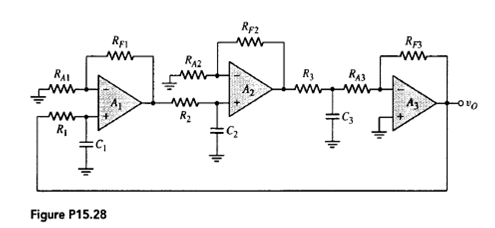Step No: 1

The given phase-shift oscillator circuit isStep No: 2

Redraw the given circuit by labeling the voltages asStep No: 3

a) Given that,
Assume thatand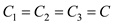and let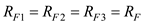.
A KCL equation at the node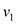yields,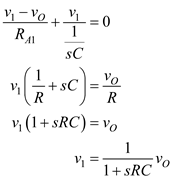Step No: 4

Amplifier gain of first op-amp isStep No: 5

Hence, the output voltages of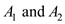op-amp are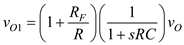…… (1)
Similarly, output of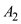op-amp is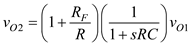…… (2)

Step No: 6

A KCL equation at the node, yields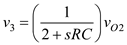…… (3)

Step No: 7

A KCL equation at the inverting terminal of aamplifier is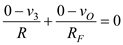…… (5)
By substituting the equation (3) in equation (4), we getStep No: 8

Substituting equation (2) in equation (5), we get

Step No: 9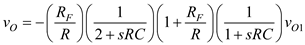…… (6)

Substituting equation (1) in equation (6), we getTo find the frequency of oscillation, set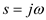and the imaginary term must be zero.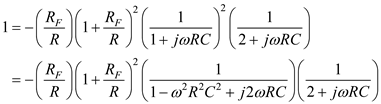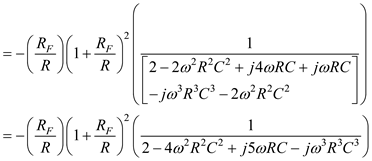Step No: 10

Consider the imaginary values then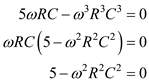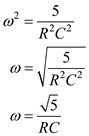Therefore, the required frequency of oscillation isStep No: 11

b) The condition for oscillation is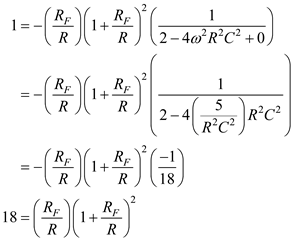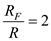from the above expression.
Hence, the required minimum magnitude of gain in each stage to oscillation is